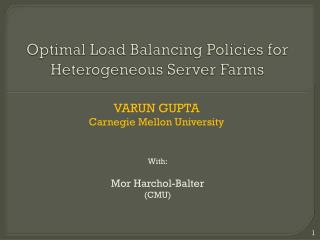# Optimal Load Balancing Policies for Heterogeneous Server Farms - PowerPoint PPT PresentationDownload PresentationOptimal Load Balancing Policies for Heterogeneous Server Farms

Optimal Load Balancing Policies for Heterogeneous Server Farms
Download Presentation## Optimal Load Balancing Policies for Heterogeneous Server Farms

- - - - - - - - - - - - - - - - - - - - - - - - - - - E N D - - - - - - - - - - - - - - - - - - - - - - - - - - -
##### Presentation Transcript

1. Optimal Load Balancing Policies for Heterogeneous Server Farms VARUN GUPTA Carnegie Mellon University With: MorHarchol-Balter (CMU)

2. Warm up GOAL: Minimize Mean Response Time E[T] K homogeneous First-Come-First-Served servers Load Balancer Poisson(λ) Knows queue lengths Not job sizes Exponentially-distributed job sizes Q: What is the optimal load balancing policy? A:Join-the-Shortest-Queue Q: Why? A: JSQ = Minimize Expected Response time of arrival

3. This Talk μ=4 GOAL: Minimize Mean Response Time E[T] K heterogeneous First-Come-First-Served servers μ=4 Load Balancer Poisson(λ) μ=1 Knows queue lengths Not job sizes Exponentially-distributed job sizes μ=1 Q: What is the optimal load balancing policy?

4. MER = Minimum Expected Response time Smart-JSQ = Join-Shortest-Queue (with smart tie breaks) μ=4 μ=4 μ=1 μ=1 μ=4 μ=4 μ=1 μ=1 Q: Which is the better policy? Q: What is the optimal policy?

5. Outline Many-servers limit: Light-traffic regime Heavy-traffic regime Complete characterization of optimal policies  First asymptotic approximations  Partial characterization of the optimal policy • Simulation Results • Effect of K • Effect of arrival rate (λ) • Effect of degree of heterogeneity

6. Many-servers light-traffic limit 4 K1 = α1K 4 Poisson(λ) 1 K2 = α2K 1

7. Many-servers light-traffic limit 4 K1 = K/3 4 Poisson(λ) 1 K2 = 2K/3 1 Q: Performance of MER • Case 2:λ> 4K/3 • Fast can nothandle λ • Can not use slow until each fast has 3 jobs ! • Case 1:λ< 4K/3 • Fast can handle λ • Arrivals find at least one fast idle •  E[T] = 1/4

8. Many-servers light-traffic limit 4 K1 = K/3 4 Poisson(λ) 1 K2 = 2K/3 1 Q: Performance of Smart-JSQ • Case 1: λ< 4K/3 • Fast can handle λ • Arrivals find at least one fast idle •  E[T] = 1/4 Case 2: λ> 4K/3 Use slow as soon as each fast has 1 job !

9. Many-servers light-traffic limit 4 K1 = K/3 4 Poisson(λ) 1 K2 = 2K/3 1 Smart-JSQbetter than MER! …but any policy which sends to slow when all fast are busy is identical in light-traffic

10. MER HYBRID (smart-JSQ+MER) Smart-JSQ μ=4 μ=4 μ=4 μ=4 μ=1 μ=1 μ=1 μ=1 smart-JSQ when some server idle μ=4 μ=4 μ=1 μ=1 MER when all busy Light-traffic  HYBRID =Smart-JSQ

11. Outline Many-servers limit: Light-traffic regime Heavy-traffic regime Complete characterization of optimal policies  First asymptotic approximations  Partial characterization of the optimal policy • Simulation Results • Effect of K • Effect of arrival rate (λ) • Effect of degree of heterogeneity

12. Many-servers heavy-traffic limit 4 K1 = α1K 4 Poisson(λ) 1 K2 = α2K 1 Analysis of JSQ for homogeneous server GOAL Analysis of policies for heterogeneous servers

13. Many-servers heavy-traffic limit 4 K1 = α1K 4 Poisson(λ) 1 K2 = α2K 1 Analysis of JSQ for homogeneous server GOAL Analysis of policies for heterogeneous servers

14. Many-servers heavy-traffic analysis for homogenous JSQ Poisson(λ) K Analysis technique: Markov chain for total jobs in system λ λ λ λ λ λ 0 1 K 3K/2 2K 2K+1 2K+2 ? = mean departure rate given 3K/2 jobs

15. N = 3K/2 K/2 Departure rate = K–1 (not K) Finding the O(1) fluctuations critical to analysis Poisson(λ) K/2 Rate = K/2 Rate = K O(1) idle queues

16. N = (1+γ)K (0<γ<1) γK Departure rate = K–(1-γ)/γ (not K) Finding the O(1) fluctuations critical to analysis Poisson(λ) (1- γ)K Rate = (1-γ)K Rate = K O(1) idle queues

17. Many-servers heavy-traffic analysis for homogenous JSQ Poisson(λ) K Analysis technique: Markov chain for total jobs in system λ λ λ λ λ λ 0 1 K 3K/2 2K 2K+1 2K+2 K K K-1 Asymptotically negligible probability mass First closed-form approx for JSQ!

18. Many-servers heavy-traffic limit 4 K1 = α1K 4 Poisson(λ) 1 K2 = α2K 1 Analysis of JSQ for homogeneous server GOAL Analysis of policies for heterogeneous servers

19. Many-servers heavy-traffic limit 4 K1 = α1K 4 Poisson(λ) 1 K2 = α2K 1 • OPT policy  maximize departure rate for each N • (preemptively) send jobs to slow servers even when they have 1 job and all fast servers have > 1 • Smart-JSQ is optimal in many-servers

20. Outline Many-servers limit: Light-traffic regime Heavy-traffic regime Complete characterization of optimal policies  First asymptotic approximations  Partial characterization of the optimal policy • Simulation Results • Effect of K • Effect of arrival rate (λ) • Effect of degree of heterogeneity

21. Many-servers light-traffic μ1=4, μ2=1, K1=100, K2=200 Smart-JSQ

22. Many-servers light-traffic μ1=4, μ2=1, K1=100, K2=200 MER Smart-JSQ

23. Many-servers light-traffic μ1=4, μ2=1, K1=100, K2=200 MER HYBRID Smart-JSQ

24. Many-servers heavy-traffic μ1=4, μ2=1, K1=100, K2=200 MER HYBRID Smart-JSQ

25. Effect of number of servers μ1=4, μ2=1, α1=1/3, α2=2/3 Smart-JSQ

26. Effect of number of servers μ1=4, μ2=1, α1=1/3, α2=2/3 MER Smart-JSQ

27. Effect of number of servers μ1=4, μ2=1, α1=1/3, α2=2/3 MER Smart-JSQ HYBRID

28. Conclusions • A new many-servers heavy-traffic scaling to analyze load balancing policies • First closed-form approx of load balancing heuristics • Choosing the right load balancer • Few servers, Small load, High heterogeneity  HYBRID • Many servers, High load, Low heterogeneity  smart-JSQ

29. Many-servers light-traffic μ1=8, μ2=1, K1=100, K2=400 MER Smart-JSQ ≈HYBRID

30. Many-servers heavy-traffic μ1=8, μ2=1, K1=100, K2=400 MER HYBRID Smart-JSQ

31. Effect of number of servers μ1=8, μ2=1, α1=1/5, α2=4/5 MER Smart-JSQ HYBRID